Question
is co-channel interference a function of transmitted power from cell site? explain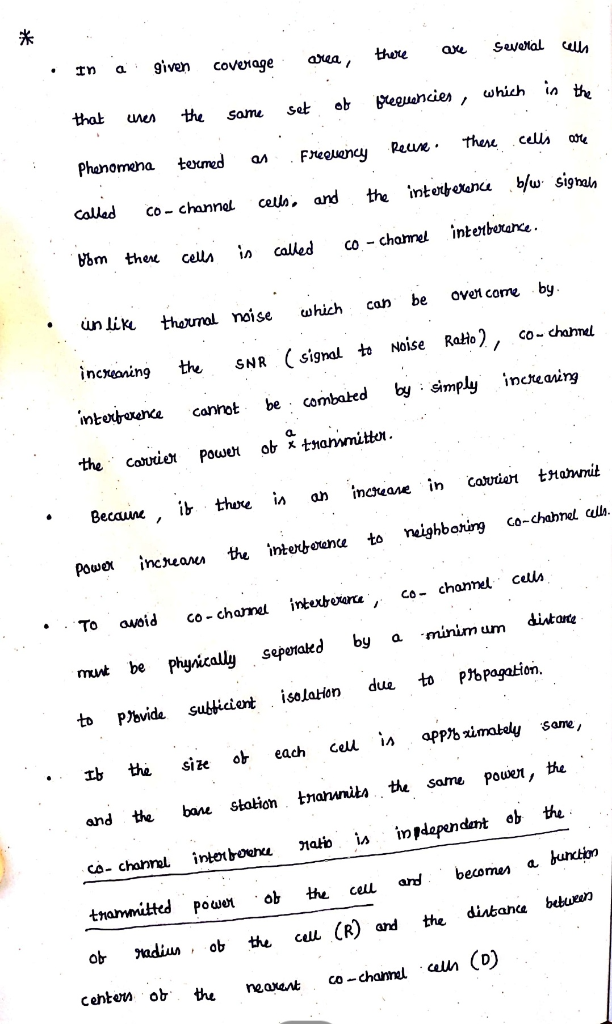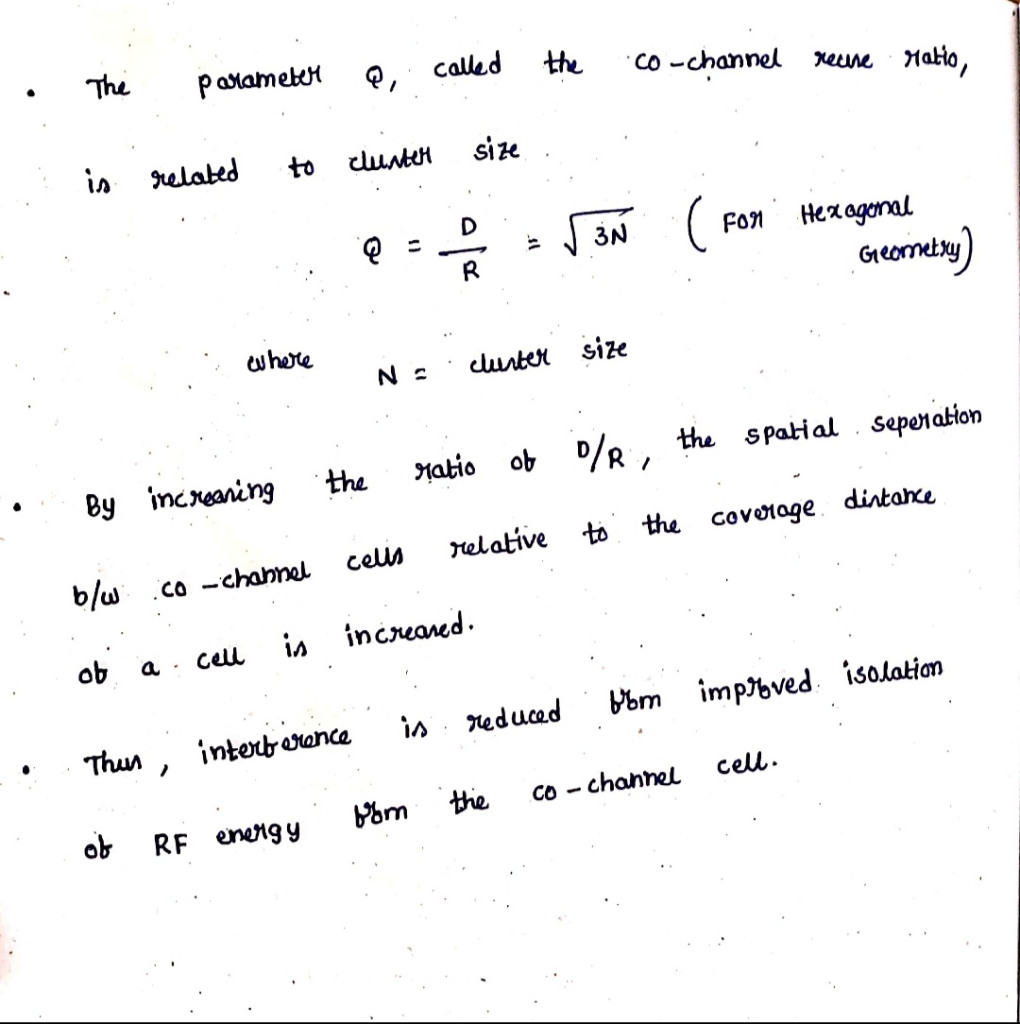#### Earn Coins

Coins can be redeemed for fabulous gifts.

Similar Homework Help Questions
• ### A radio channel has a bandwidth of 7MHz, and transmitted signal power equal to the noise...

A radio channel has a bandwidth of 7MHz, and transmitted signal power equal to the noise power. Calculate the capacity of the channel

• ### describe the process of the modulation and parameter setting, and co-channel interference.

describe the process of the modulation and parameter setting, and co-channel interference.

• ### 4) At the rate of 10 bps binary data are transmitted in a channel. Power spectral...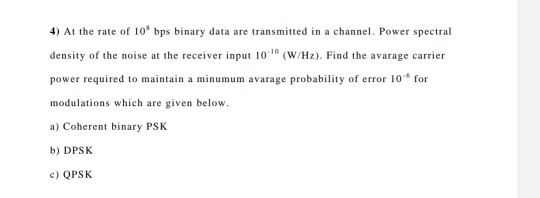4) At the rate of 10 bps binary data are transmitted in a channel. Power spectral density of the noise at the receiver input 10 (W/Hz). Find the avarage carrier power required to maintain a minumum avarage probability of error 10 for modulations which are given below. a) Coherent binary PSK b) DPSK c) QPSK

• ### Q1. A DSB-SC signal is transmitted over a noisy channel with power spectral density of noise...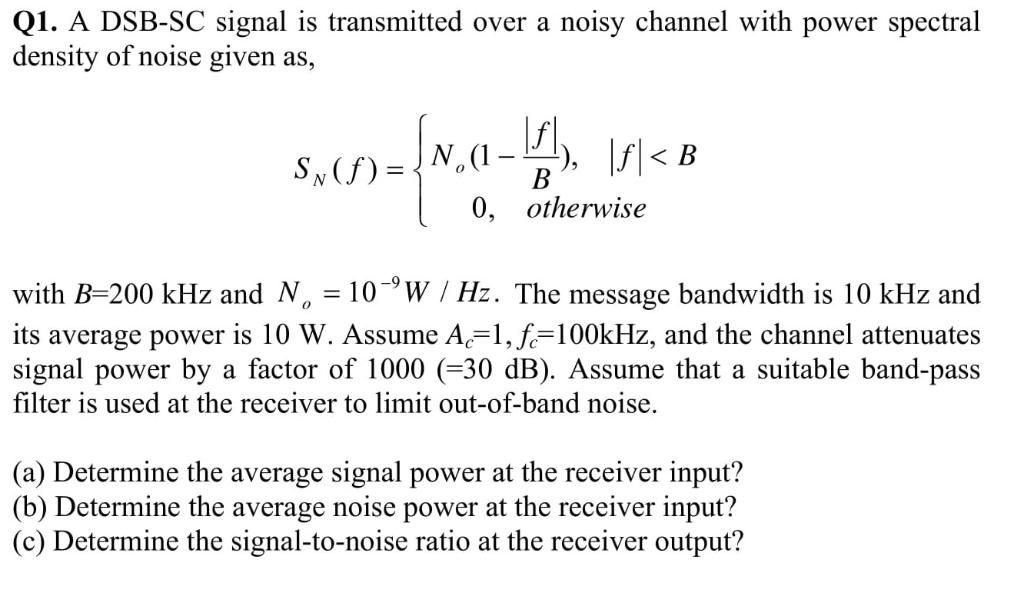Q1. A DSB-SC signal is transmitted over a noisy channel with power spectral density of noise given as, 0, otherwise with B-200 kHz and N 10W Hz. The message bandwidth is 10 kHz and its average power is 10 W. Assume A,-1, 100kHz, and the channel attenuates signal power by a factor of 1000 (-30 dB). Assume that a suitable band-pass filter is used at the receiver to limit out-of-band noise. (a) Determine the average signal power at the receiver...

• ### The time-variant transfer function of a WSSUS channel is given by: (a) Does the channel exhibit f...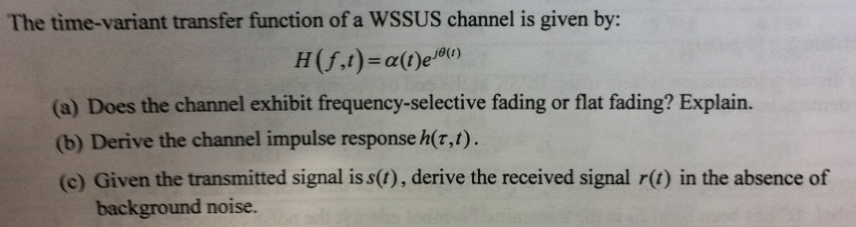The time-variant transfer function of a WSSUS channel is given by: (a) Does the channel exhibit frequency-selective fading or flat fading? Explain. (b) Derive the channel impulse response h(r,t). (c) Given the transmitted signal is s(), derive the received signal r) in the absence of background noise. The time-variant transfer function of a WSSUS channel is given by: (a) Does the channel exhibit frequency-selective fading or flat fading? Explain. (b) Derive the channel impulse response h(r,t). (c) Given the transmitted...

• ### B4. A cellular system uses 120° sectoring, i.e. each cell is split into three equal parts. (i) Find the number of interfering co-channel cells Co) for the following ls sd cluster sizes N-3, and N...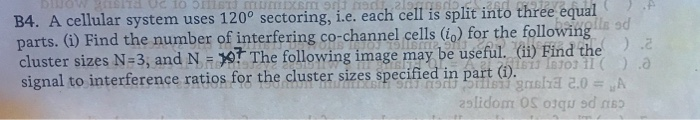B4. A cellular system uses 120° sectoring, i.e. each cell is split into three equal parts. (i) Find the number of interfering co-channel cells Co) for the following ls sd cluster sizes N-3, and N, The following image may be useful. (Gi) Find the signal to interference ratios for the dluster sizes specifed in partnaba 20- A B4. A cellular system uses 120° sectoring, i.e. each cell is split into three equal parts. (i) Find the number of interfering co-channel...

• ### Write the transmitted signal of QPSK ofs(t) for i-1, 2, 3, 4, and 0 t 2) Explain why this modulation scheme is called QPSK 3) If the transmitted signals are sent over an AWGN channel, what is the opt...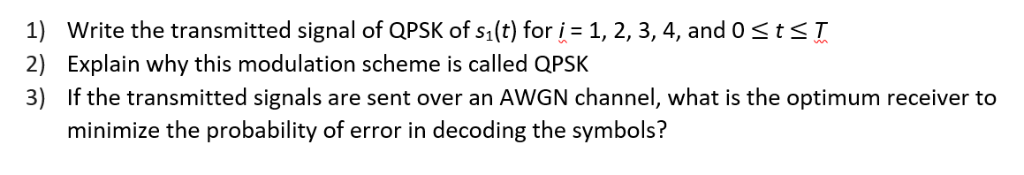Write the transmitted signal of QPSK ofs(t) for i-1, 2, 3, 4, and 0 t 2) Explain why this modulation scheme is called QPSK 3) If the transmitted signals are sent over an AWGN channel, what is the optimum receiver to minimize the probability of error in decoding the symbols? Write the transmitted signal of QPSK ofs(t) for i-1, 2, 3, 4, and 0 t 2) Explain why this modulation scheme is called QPSK 3) If the transmitted signals are...

• ### The following is the channel frequency assignment for a 12-channel AM radio system: (c) Channel No Fcy 1240 kHz 1370 kHz 500 kHz 1630 kHz Channel No.Frequency 2280 kHz 2410 kHz 2540 kHz 2670 kHz Chan...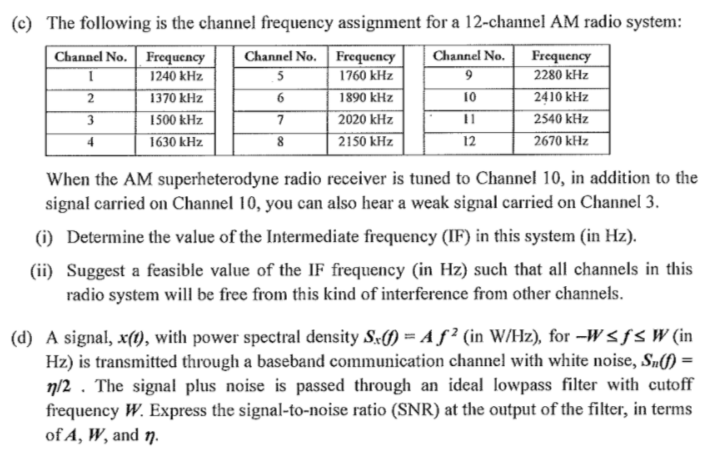The following is the channel frequency assignment for a 12-channel AM radio system: (c) Channel No Fcy 1240 kHz 1370 kHz 500 kHz 1630 kHz Channel No.Frequency 2280 kHz 2410 kHz 2540 kHz 2670 kHz Channel No. Freqency 1760 kHz 1890 kHz 2020 kHz 2150 kHz 10 2 12 When the AM superheterodyne radio receiver is tuned to Channel 10, in addition to the signal carried on Channel 10, you can also hear a weak signal carried on Channel3 (i)...

• ### Problem 3. Obtain the expression for the half-power frequencies (-3 dB) for second order Sullen-Key band-pass filter with transfer function: co and network function: a。 ώρ Explain the meaning of...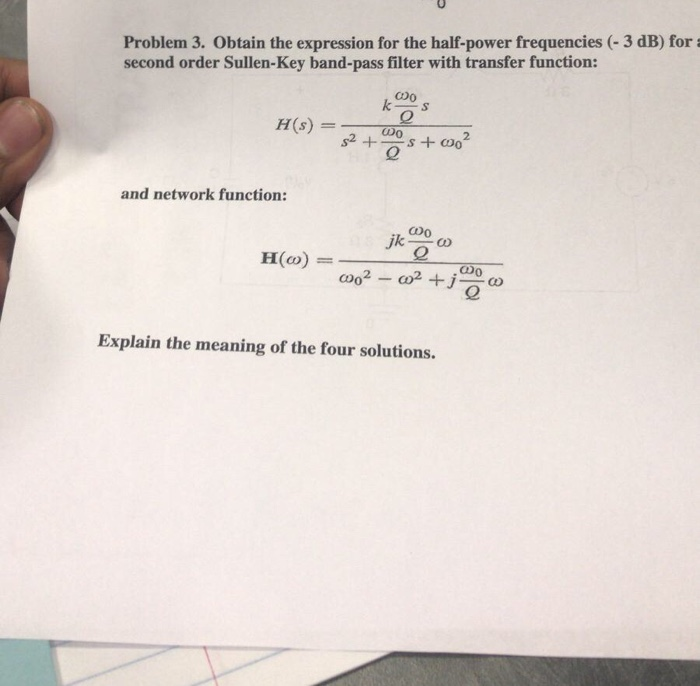Problem 3. Obtain the expression for the half-power frequencies (-3 dB) for second order Sullen-Key band-pass filter with transfer function: co and network function: a。 ώρ Explain the meaning of the four solutions. Problem 3. Obtain the expression for the half-power frequencies (-3 dB) for second order Sullen-Key band-pass filter with transfer function: co and network function: a。 ώρ Explain the meaning of the four solutions.

• ### All of 1 A certain channel has been isolated from a cell membrane, that allows cyanide...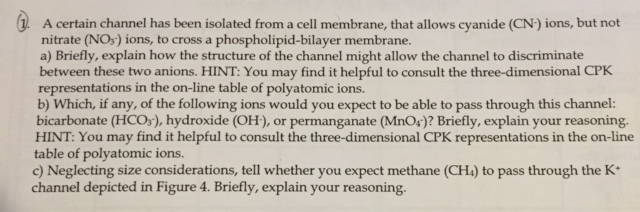All of 1 A certain channel has been isolated from a cell membrane, that allows cyanide (CN-) ions, but not nitrate (NO3) ions, to cross a phospholipid-bilayer membrane. a) Briefly, explain how the structure of the channel might allow the channel to discriminate between these two anions. HINT: You may find it helpful to consult the three-dimensional CPK representations in the on-line table of polyatomic ions. b) Which, if any, of the following ions would you expect to be able...